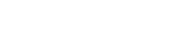# client.as.number

Available inall subroutines.

Autonomous system (AS) number.

The `INTEGER` type in VCL is wide enough to support the full range of 32-bit AS numbers.

## Converting to asdot+ format

Formatting these numbers to base 10 (e.g., by implicit conversion to a `STRING` type) will give an `asplain` representation of the number, which is just its base 10 representation.

RFC 5396 introduces the `asdot+` format, which represents a 32-bit AS number as two 16-bit parts. The following VCL illustrates constructing an `asdot+` formatted number:

``````sub client_asn_dotplus STRING {  declare local var.hi INTEGER;  declare local var.lo INTEGER;  declare local var.s STRING;
set var.hi = client.as.number;  set var.hi >>= 16;  set var.lo = client.as.number;  set var.lo &= 0xFFFF;
set var.s = client.as.number ": " var.hi "." var.lo;
return var.s;}``````

## Examples

The 32-bit AS number 65550 (reserved by RFC 5398 for documentation use) is rendered as `1.14`.

Several ranges of AS numbers are reserved for various purposes. The following VCL fragment illustrates categorizing AS numbers into these ranges:

``````sub client_asn_category STRING {  declare local var.asn_category STRING;
if (client.as.number < 0 || client.as.number > 0xFFFFFFFF) {    set var.asn_category = "invalid";  } else if (client.as.number == 0) {    set var.asn_category = "reserved"; # RFC 1930  } else if (client.as.number <= 23455) {    set var.asn_category = "public";  } else if (client.as.number == 23456) {    set var.asn_category = "transition"; # RFC 6793  } else if (client.as.number <= 64534) {    set var.asn_category = "public";  } else if (client.as.number <= 64495) {    set var.asn_category = "reserved";  } else if (client.as.number <= 64511) {    set var.asn_category = "documentation"; # RFC 5398  } else if (client.as.number <= 65534) {    set var.asn_category = "private";  } else if (client.as.number == 65535) {    set var.asn_category = "reserved";  } else if (client.as.number <= 65551) {    set var.asn_category = "documentation"; # RFC 4893, RFC 5398  } else if (client.as.number <= 131071) {    set var.asn_category = "reserved";  } else if (client.as.number <= 4199999999) {    set var.asn_category = "public";  } else if (client.as.number <= 4294967294) {    set var.asn_category = "private"; # RFC 6996  } else if (client.as.number == 4294967295) {    set var.asn_category = "reserved";  } else {    set var.asn_category = "unknown";  }
return var.asn_category;}``````

## Try it out

`client.as.number` is used in the following code examples. Examples apply VCL to real-world use cases and can be deployed as they are, or adapted for your own service. See the full list of code examples for more inspiration.

Click RUN on a sample below to provision a Fastly service, execute the code on Fastly, and see how the function behaves.

## Comprehensive logging

Fastly offers a myriad of different variables that you can log. See and test a large collection here.

BETA